# Pandas apply, map and applymap

In this post we will see how to apply a function along the axis of a dataframe using apply and applymap and how to map the values of a Series from one domain to another using map

## When to use apply, applymap and map?

Apply: It is used when you want to apply a function along the axis of a dataframe, it accepts a Series whose index is either column (axis=0) or row (axis=1). For example: df.apply(np.square), it will give a dataframe with number squared

applymap: It is used for element wise operation across one or more rows and columns of a dataframe. It has been optimized and some cases work faster than apply but it’s good to compare it with apply before going for any heavier operation . Example: df.applymap(np.square), it will give a dataframe with number squared

map: It can be used only for a Series object and helps to substitutes the series value from the lookup dictionary, Series or a function and missing value will be substituted as NaN. Since it works only with series or dictionary so you can expect a better and optimized performance. Example: df[‘Col1’].map({‘Trenton’:’New Jersey’, ‘NYC’:’New York’, ‘Los Angeles’:’California’})

## How to use Pandas apply?

First create a dataframe with three rows a,b and c and indexes A1,B1,C1

### Create Dataframe

``````import pandas as pd
import numpy as np
df = pd.DataFrame({'a' : [ 10,20,30],'b' : [5,10,15],'c' : [10,100,1000]},index=['A1','B1','C1'])
df
``````

Ouput: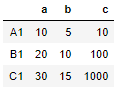### Define functions

We will define two functions to apply to this dataframe, function multiply_by_2 will be applied across the column and multiply_col1_col2 will be applied across the rows of dataframe

``````def multiply_by_2(col):
return col*2

def multiply_col1_col2(col):
return col['a']*col['b']
``````

### Apply function across dataframe columns(axis=0)

Now apply the function multiply_by_2 across the columns, so by default the value of axis=0, so we have to just pass the function without axis parameter

``````df.apply(multiply_by_2)
``````

Output:

All the cell values are doubled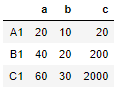### Apply function across dataframe rows(axis=1)

Now apply the function multiply_col1_col2 across the rows of the dataframe. Here we have set the axis parameter as 1 (axis=1)

``````df.apply(multiply_col1_col2,axis=1)
``````

It will return a series object with values obtained by multiplying col1 and col2 with the same indexes

``````A1     50
B1    200
C1    450
dtype: int64
``````

### Create a new Column col1xcol2 with the above series

Now we will create a new column using the above Series called as ‘Col1XCol2”

``````df['col1Xcol2'] = df.apply(multiply_col1_col2,axis=1)
``````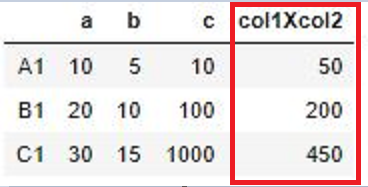## Pandas apply function with Result_type parameter

It’s a parameter set to {expand, reduce or broadcast} to get the desired type of result. the default value is None

In the above scenario if result_type is set to broadcast then the output will be a dataframe substituted by the Col1xCol2 value

``````df.apply(multiply_col1_col2,axis=1,result_type='broadcast')
``````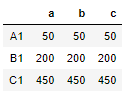The results is broadcasted to the original shape of the frame, the original index and columns is retained

To understand result_type as expand and reduce we will first create a function that returns a list value

``````def multi_and_list(col):
return [col['a']*2,col['b']*2,col['c']*2]
``````

Now apply this function across the dataframe column with result_type as expand

``````df.apply(multi_and_list,axis=1,result_type='expand')
``````

if result_type is set as expand then It returns a dataframe though the function returns a list.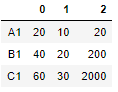result_type reduce is just opposite of expand and returns a Series if possible rather than expanding list-like results

## How to use lambda with apply?

you can also use lambda expression with pandas apply function.

We will multiply the values at Col1 and Col2 as shown above using the lambda function. Since we have to apply this for each row so we will use axis=1

``````df.apply(lambda x: x['a']*x['b'],axis=1)
``````

Output:

A1 50 B1 200 C1 450 dtype: int64

## Pandas apply function with arguments

Many a times we have to pass an additional argument to a function and it’s a good news that You can also pass a positional argument and keyword argument to apply function.

### Create a Function with argument

This function calculates the haversine distance between two geo-coordinates and takes a series of origin and dest lat and long and an additional argument radius(rad)

In the following section we will see how to pass this radius as an argument

``````from math import radians, cos, sin, asin, sqrt

"""
Calculate the great circle distance between two points
on the earth (specified in decimal degrees)
"""
# convert decimal degrees to radians
lon1, lat1, lon2, lat2 = map(radians, [row['dest_long'], row['dest_lat'],row['orig_long'], row['orig_lat']])

# haversine formula
dlon = lon2 - lon1
dlat = lat2 - lat1
a = sin(dlat/2)**2 + cos(lat1) * cos(lat2) * sin(dlon/2)**2
c = 2 * asin(sqrt(a))
r = rad # Radius of earth in kilometers. Use 3956 for miles
return c * r
``````

### Create dataframe with origin and destination city latitude and longitude

``````df_coords = pd.DataFrame({'orig_city':['New York','Charlotte','Boston','Bridgewater'],
'orig_lat':[40.7128,35.2271,42.36,40.594],
'orig_long':[74.006,80.843,71.0589,74.604],
'dest_city':['Trenton','Texas','Sunnyvale','San Jose'],
'dest_lat':[40.2206,31.9686,37.3688,37.3382],
'dest_long':[40.2206,31.9686,37.3688,37.3382]})
df_coords
``````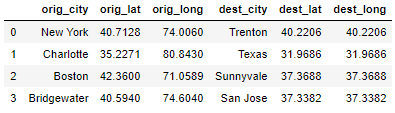## Apply function with arguments

Now we will find haversine distance between origin and destination city in the above dataframe. So we will apply the haversine function defined above using the apply function.

In haversine function above rad is a required argument and the dataframe doesn’t have any radius column.

We will pass the radius values as args=(3956,) in the apply function as a positional argument. to calculate distance in miles

``````df['haversine_dist]=df_coords.apply(haversine,axis=1,args=(3956,))
``````

We will add the calculated haversine distance as a new column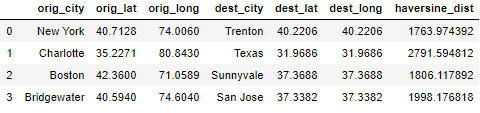## Apply function with keyword arguments (kwds)

We will pass the radius values as keyword argument as rad=3956 in the apply function to calculate distance in kilometer

``````df_coords['orig_dest_haver_dist']=df_coords.apply(haversine,axis=1,rad=3956)
df_coords
``````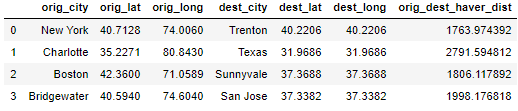## How to use Pandas applymap?

As defined above, it is used for element wise operation of a dataframe and a scalar value is returned for every elements

We will square each number in the above dataframe using lambda expression with applymap function

``````df.applymap(lambda x: x**2)
``````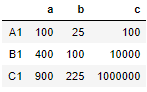There are more vectorized way of doing this operation is available like df *2 which is much faster and optimized

## How to use Pandas map?

Maps are used to map or substitute a value from a lookup table i.e. a dictionary, function or a series here.

I would suggest to read this detailed post on how to use pandas map function

Tags:

Categories:

Updated: